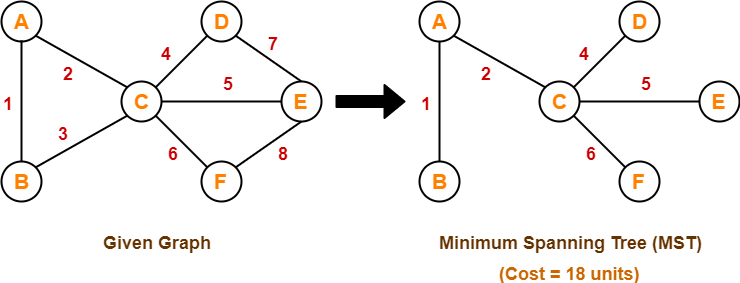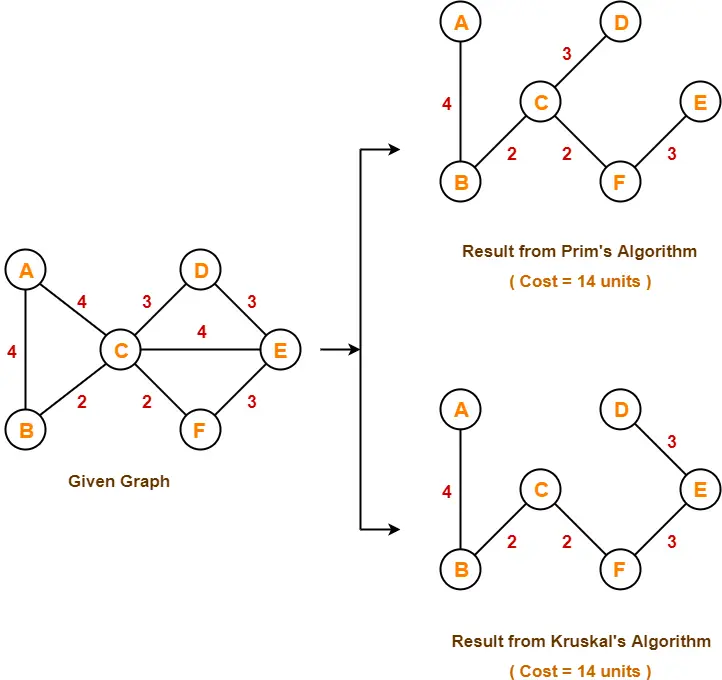# Difference Between Prim’s and Kruskal’s Algorithm

## Prim’s and Kruskal’s Algorithms-

Before you go through this article, make sure that you have gone through the previous articles on Prim’s Algorithm & Kruskal’s Algorithm.

We have discussed-

• Prim’s and Kruskal’s Algorithm are the famous greedy algorithms.
• They are used for finding the Minimum Spanning Tree (MST) of a given graph.
• To apply these algorithms, the given graph must be weighted, connected and undirected.

Some important concepts based on them are-

## Concept-01:

If all the edge weights are distinct, then both the algorithms are guaranteed to find the same MST.

### Example-

Consider the following example-Here, both the algorithms on the above given graph produces the same MST as shown.

## Concept-02:

• If all the edge weights are not distinct, then both the algorithms may not always produce the same MST.
• However, cost of both the MSTs would always be same in both the cases.

### Example-

Consider the following example-Here, both the algorithms on the above given graph produces different MSTs as shown but the cost is same in both the cases.

## Concept-03:

Kruskal’s Algorithm is preferred when-

• The graph is sparse.
• There are less number of edges in the graph like E = O(V)
• The edges are already sorted or can be sorted in linear time.

Prim’s Algorithm is preferred when-

• The graph is dense.
• There are large number of edges in the graph like E = O(V2).

## Concept-04:

Difference between Prim’s Algorithm and Kruskal’s Algorithm-

 Prim’s Algorithm Kruskal’s Algorithm The tree that we are making or growing always remains connected. The tree that we are making or growing usually remains disconnected. Prim’s Algorithm grows a solution from a random vertex by adding the next cheapest vertex to the existing tree. Kruskal’s Algorithm grows a solution from the cheapest edge by adding the next cheapest edge to the existing tree / forest. Prim’s Algorithm is faster for dense graphs. Kruskal’s Algorithm is faster for sparse graphs.

To gain better understanding about Difference between Prim’s and Kruskal’s Algorithm,

Watch this Video Lecture

Next Article- Linear Search

Get more notes and other study material of Design and Analysis of Algorithms.

Watch video lectures by visiting our YouTube channel LearnVidFun.

SummaryArticle Name
Difference Between Prim's and Kruskal's Algorithm
Description
Difference Between Prim's and Kruskal's Algorithm- In Prim's Algorithm, the tree that we are growing always remains connected while in Kruskal's Algorithm, the tree that we are growing usually remains disconnected.
Author
Publisher Name
Gate Vidyalay
Publisher Logo